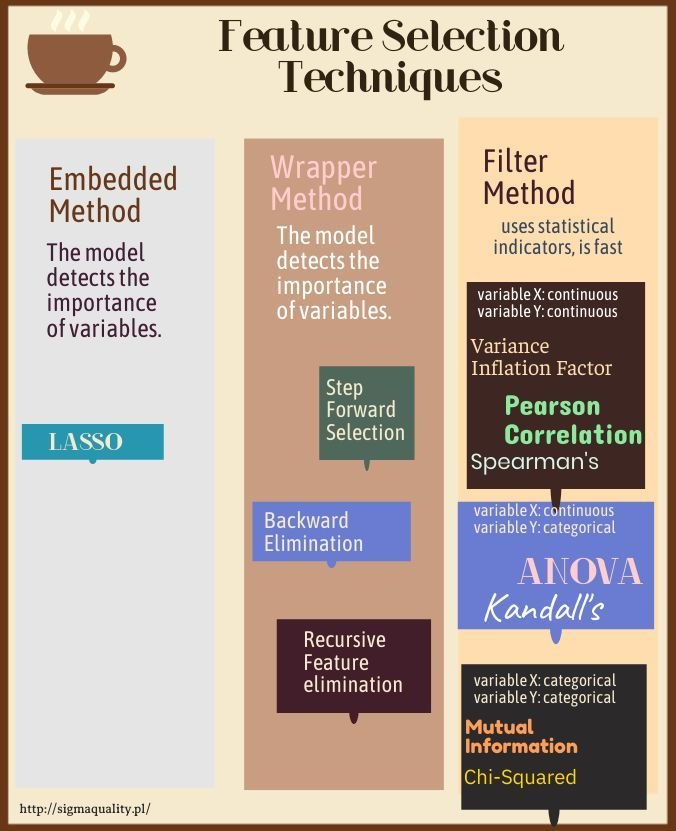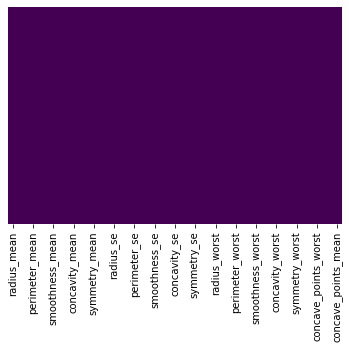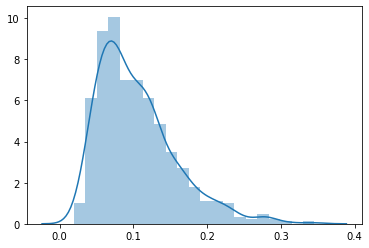# Feature Selection Techniques [numerical result] – Step Forward Selection

300320201248Forward selection is an iterative method in which we start with no function in the model. In each iteration, we add a function that best improves our model until adding a new variable improves the model’s performance.
In :
import numpy as np
import pandas as pd
import seaborn as sns
import matplotlib.pyplot as plt
from sklearn.preprocessing import LabelEncoder, OneHotEncoder
import warnings
warnings.filterwarnings("ignore")
from sklearn.model_selection import train_test_split
from sklearn.svm import SVC
from sklearn.metrics import confusion_matrix
np.random.seed(123)

In :
##  colorful prints
def black(text):
print('33[30m', text, '33[0m', sep='')
def red(text):
print('33[31m', text, '33[0m', sep='')
def green(text):
print('33[32m', text, '33[0m', sep='')
def yellow(text):
print('33[33m', text, '33[0m', sep='')
def blue(text):
print('33[34m', text, '33[0m', sep='')
def magenta(text):
print('33[35m', text, '33[0m', sep='')
def cyan(text):
print('33[36m', text, '33[0m', sep='')
def gray(text):
print('33[90m', text, '33[0m', sep='')

In :
df = pd.read_csv ('/home/wojciech/Pulpit/6/Breast_Cancer_Wisconsin.csv')
green(df.shape)

(569, 33)

Out:
id diagnosis radius_mean texture_mean perimeter_mean area_mean smoothness_mean compactness_mean concavity_mean concave points_mean texture_worst perimeter_worst area_worst smoothness_worst compactness_worst concavity_worst concave points_worst symmetry_worst fractal_dimension_worst Unnamed: 32
0 842302 M 17.99 10.38 122.8 1001.0 0.11840 0.27760 0.3001 0.14710 17.33 184.6 2019.0 0.1622 0.6656 0.7119 0.2654 0.4601 0.11890 NaN
1 842517 M 20.57 17.77 132.9 1326.0 0.08474 0.07864 0.0869 0.07017 23.41 158.8 1956.0 0.1238 0.1866 0.2416 0.1860 0.2750 0.08902 NaN
2 84300903 M 19.69 21.25 130.0 1203.0 0.10960 0.15990 0.1974 0.12790 25.53 152.5 1709.0 0.1444 0.4245 0.4504 0.2430 0.3613 0.08758 NaN

3 rows × 33 columns

### Deleting unneeded columns¶

In :
df['concave_points_worst'] = df['concave points_worst']
df['concave_points_se'] = df['concave points_se']
df['concave_points_mean'] = df['concave points_mean']

del df['Unnamed: 32']
del df['diagnosis']
del df['id']

In :
df.isnull().sum()

Out:
radius_mean                0
texture_mean               0
perimeter_mean             0
area_mean                  0
smoothness_mean            0
compactness_mean           0
concavity_mean             0
concave points_mean        0
symmetry_mean              0
fractal_dimension_mean     0
texture_se                 0
perimeter_se               0
area_se                    0
smoothness_se              0
compactness_se             0
concavity_se               0
concave points_se          0
symmetry_se                0
fractal_dimension_se       0
texture_worst              0
perimeter_worst            0
area_worst                 0
smoothness_worst           0
compactness_worst          0
concavity_worst            0
concave points_worst       0
symmetry_worst             0
fractal_dimension_worst    0
concave_points_worst       0
concave_points_se          0
concave_points_mean        0
dtype: int64
In :
import seaborn as sns

sns.heatmap(df.isnull(),yticklabels=False,cbar=False,cmap='viridis')

Out:
<matplotlib.axes._subplots.AxesSubplot at 0x7f87ef7ed310>### Deletes duplicates¶

there were no duplicates

In :
green(df.shape)
df.drop_duplicates(keep='first', inplace=True)
blue(df.shape)

(569, 33)
(569, 33)

In :
blue(df.dtypes)

radius_mean                float64
texture_mean               float64
perimeter_mean             float64
area_mean                  float64
smoothness_mean            float64
compactness_mean           float64
concavity_mean             float64
concave points_mean        float64
symmetry_mean              float64
fractal_dimension_mean     float64
texture_se                 float64
perimeter_se               float64
area_se                    float64
smoothness_se              float64
compactness_se             float64
concavity_se               float64
concave points_se          float64
symmetry_se                float64
fractal_dimension_se       float64
texture_worst              float64
perimeter_worst            float64
area_worst                 float64
smoothness_worst           float64
compactness_worst          float64
concavity_worst            float64
concave points_worst       float64
symmetry_worst             float64
fractal_dimension_worst    float64
concave_points_worst       float64
concave_points_se          float64
concave_points_mean        float64
dtype: object

In :
df.columns

Out:
Index(['radius_mean', 'texture_mean', 'perimeter_mean', 'area_mean',
'smoothness_mean', 'compactness_mean', 'concavity_mean',
'concave points_mean', 'symmetry_mean', 'fractal_dimension_mean',
'compactness_se', 'concavity_se', 'concave points_se', 'symmetry_se',
'perimeter_worst', 'area_worst', 'smoothness_worst',
'compactness_worst', 'concavity_worst', 'concave points_worst',
'symmetry_worst', 'fractal_dimension_worst', 'concave_points_worst',
'concave_points_se', 'concave_points_mean'],
dtype='object')

### We choose the continuous variable – compactness_mean¶

In :
print('max:',df['compactness_mean'].max())
print('min:',df['compactness_mean'].min())

sns.distplot(np.array(df['compactness_mean']))

max: 0.3454
min: 0.01938

Out:
<matplotlib.axes._subplots.AxesSubplot at 0x7f87ec3f3050># Step Forward Selection¶

In :
X = df.drop('compactness_mean', axis=1)
y = df['compactness_mean']

from sklearn.model_selection import train_test_split

X_train, X_test, y_train, y_test = train_test_split(X, y, test_size=0.20, random_state=123)
# Jeżeli się rzuca wtedy wycinamy stratify=y.


I specify how many programs should indicate the best variables:

In :
k_features = 16

In :
from sklearn.linear_model import LinearRegression
from mlxtend.feature_selection import SequentialFeatureSelector as sfs

LR = LinearRegression()

sfs1 = sfs(LR,k_features = k_features, forward=True, floating=False, scoring='r2',verbose=2,cv=5)
sfs1 = sfs1.fit(X_train,y_train)

[Parallel(n_jobs=1)]: Using backend SequentialBackend with 1 concurrent workers.
[Parallel(n_jobs=1)]: Done   1 out of   1 | elapsed:    0.0s remaining:    0.0s
[Parallel(n_jobs=1)]: Done  32 out of  32 | elapsed:    0.3s finished

[2020-03-30 12:43:15] Features: 1/16 -- score: 0.7605648031784296[Parallel(n_jobs=1)]: Using backend SequentialBackend with 1 concurrent workers.
[Parallel(n_jobs=1)]: Done   1 out of   1 | elapsed:    0.0s remaining:    0.0s
[Parallel(n_jobs=1)]: Done  31 out of  31 | elapsed:    0.3s finished

[2020-03-30 12:43:15] Features: 2/16 -- score: 0.8592594816229919[Parallel(n_jobs=1)]: Using backend SequentialBackend with 1 concurrent workers.
[Parallel(n_jobs=1)]: Done   1 out of   1 | elapsed:    0.0s remaining:    0.0s
[Parallel(n_jobs=1)]: Done  30 out of  30 | elapsed:    0.2s finished

[2020-03-30 12:43:15] Features: 3/16 -- score: 0.9171881609890725[Parallel(n_jobs=1)]: Using backend SequentialBackend with 1 concurrent workers.
[Parallel(n_jobs=1)]: Done   1 out of   1 | elapsed:    0.0s remaining:    0.0s
[Parallel(n_jobs=1)]: Done  29 out of  29 | elapsed:    0.3s finished

[2020-03-30 12:43:16] Features: 4/16 -- score: 0.9392541495763911[Parallel(n_jobs=1)]: Using backend SequentialBackend with 1 concurrent workers.
[Parallel(n_jobs=1)]: Done   1 out of   1 | elapsed:    0.0s remaining:    0.0s
[Parallel(n_jobs=1)]: Done  28 out of  28 | elapsed:    0.3s finished

[2020-03-30 12:43:16] Features: 5/16 -- score: 0.9483152571280057[Parallel(n_jobs=1)]: Using backend SequentialBackend with 1 concurrent workers.
[Parallel(n_jobs=1)]: Done   1 out of   1 | elapsed:    0.0s remaining:    0.0s
[Parallel(n_jobs=1)]: Done  27 out of  27 | elapsed:    0.2s finished

[2020-03-30 12:43:16] Features: 6/16 -- score: 0.95545376115284[Parallel(n_jobs=1)]: Using backend SequentialBackend with 1 concurrent workers.
[Parallel(n_jobs=1)]: Done   1 out of   1 | elapsed:    0.0s remaining:    0.0s
[Parallel(n_jobs=1)]: Done  26 out of  26 | elapsed:    0.2s finished

[2020-03-30 12:43:16] Features: 7/16 -- score: 0.9575365106130604[Parallel(n_jobs=1)]: Using backend SequentialBackend with 1 concurrent workers.
[Parallel(n_jobs=1)]: Done   1 out of   1 | elapsed:    0.0s remaining:    0.0s
[Parallel(n_jobs=1)]: Done  25 out of  25 | elapsed:    0.2s finished

[2020-03-30 12:43:17] Features: 8/16 -- score: 0.9679393948794752[Parallel(n_jobs=1)]: Using backend SequentialBackend with 1 concurrent workers.
[Parallel(n_jobs=1)]: Done   1 out of   1 | elapsed:    0.0s remaining:    0.0s
[Parallel(n_jobs=1)]: Done  24 out of  24 | elapsed:    0.2s finished

[2020-03-30 12:43:17] Features: 9/16 -- score: 0.9722927912279392[Parallel(n_jobs=1)]: Using backend SequentialBackend with 1 concurrent workers.
[Parallel(n_jobs=1)]: Done   1 out of   1 | elapsed:    0.0s remaining:    0.0s
[Parallel(n_jobs=1)]: Done  23 out of  23 | elapsed:    0.2s finished

[2020-03-30 12:43:17] Features: 10/16 -- score: 0.9734667931156942[Parallel(n_jobs=1)]: Using backend SequentialBackend with 1 concurrent workers.
[Parallel(n_jobs=1)]: Done   1 out of   1 | elapsed:    0.0s remaining:    0.0s
[Parallel(n_jobs=1)]: Done  22 out of  22 | elapsed:    0.2s finished

[2020-03-30 12:43:17] Features: 11/16 -- score: 0.9743145044074704[Parallel(n_jobs=1)]: Using backend SequentialBackend with 1 concurrent workers.
[Parallel(n_jobs=1)]: Done   1 out of   1 | elapsed:    0.0s remaining:    0.0s
[Parallel(n_jobs=1)]: Done  21 out of  21 | elapsed:    0.2s finished

[2020-03-30 12:43:17] Features: 12/16 -- score: 0.9751371831838199[Parallel(n_jobs=1)]: Using backend SequentialBackend with 1 concurrent workers.
[Parallel(n_jobs=1)]: Done   1 out of   1 | elapsed:    0.0s remaining:    0.0s
[Parallel(n_jobs=1)]: Done  20 out of  20 | elapsed:    0.2s finished

[2020-03-30 12:43:18] Features: 13/16 -- score: 0.9753888664795454[Parallel(n_jobs=1)]: Using backend SequentialBackend with 1 concurrent workers.
[Parallel(n_jobs=1)]: Done   1 out of   1 | elapsed:    0.0s remaining:    0.0s
[Parallel(n_jobs=1)]: Done  19 out of  19 | elapsed:    0.2s finished

[2020-03-30 12:43:18] Features: 14/16 -- score: 0.9756613892479665[Parallel(n_jobs=1)]: Using backend SequentialBackend with 1 concurrent workers.
[Parallel(n_jobs=1)]: Done   1 out of   1 | elapsed:    0.0s remaining:    0.0s
[Parallel(n_jobs=1)]: Done  18 out of  18 | elapsed:    0.2s finished

[2020-03-30 12:43:18] Features: 15/16 -- score: 0.9758538991452695[Parallel(n_jobs=1)]: Using backend SequentialBackend with 1 concurrent workers.
[Parallel(n_jobs=1)]: Done   1 out of   1 | elapsed:    0.0s remaining:    0.0s
[Parallel(n_jobs=1)]: Done  17 out of  17 | elapsed:    0.2s finished

[2020-03-30 12:43:18] Features: 16/16 -- score: 0.9768921740889114
In :
feat_cols =list(sfs1.k_feature_idx_)
print(feat_cols)

[0, 2, 3, 4, 5, 6, 7, 8, 14, 18, 19, 21, 24, 25, 27, 28]

In :
X.columns

Out:
Index(['radius_mean', 'texture_mean', 'perimeter_mean', 'area_mean',
'smoothness_mean', 'concavity_mean', 'concave points_mean',
'perimeter_se', 'area_se', 'smoothness_se', 'compactness_se',
'concavity_se', 'concave points_se', 'symmetry_se',
'perimeter_worst', 'area_worst', 'smoothness_worst',
'compactness_worst', 'concavity_worst', 'concave points_worst',
'symmetry_worst', 'fractal_dimension_worst', 'concave_points_worst',
'concave_points_se', 'concave_points_mean'],
dtype='object')
In :
new_cols = df.columns[feat_cols]
new_cols

Out:
Index(['radius_mean', 'perimeter_mean', 'area_mean', 'smoothness_mean',
'compactness_mean', 'concavity_mean', 'concave points_mean',
'symmetry_mean', 'smoothness_se', 'symmetry_se', 'fractal_dimension_se',
'texture_worst', 'smoothness_worst', 'compactness_worst',
'concave points_worst', 'symmetry_worst'],
dtype='object')

#### I create a dataset with reduced columns.

In :
df2 = df[new_cols]

Out:
radius_mean perimeter_mean area_mean smoothness_mean compactness_mean concavity_mean concave points_mean symmetry_mean smoothness_se symmetry_se fractal_dimension_se texture_worst smoothness_worst compactness_worst concave points_worst symmetry_worst
0 17.99 122.8 1001.0 0.11840 0.27760 0.3001 0.14710 0.2419 0.006399 0.03003 0.006193 17.33 0.1622 0.6656 0.2654 0.4601
1 20.57 132.9 1326.0 0.08474 0.07864 0.0869 0.07017 0.1812 0.005225 0.01389 0.003532 23.41 0.1238 0.1866 0.1860 0.2750
2 19.69 130.0 1203.0 0.10960 0.15990 0.1974 0.12790 0.2069 0.006150 0.02250 0.004571 25.53 0.1444 0.4245 0.2430 0.3613

### OLS linear regression model for variables before reduction¶

In :
blue(df.shape)

(569, 33)

In :
X1 = df.drop('compactness_mean', axis=1)
y1 = df['compactness_mean']

In :
from statsmodels.formula.api import ols
import statsmodels.api as sm

model_fit = model.fit()

print('R2: %.6f' % model_fit.rsquared)
#blue(model_fit.summary())

R2: 0.980200


### OLS linear regression model for variables after reduction¶

In :
X2 = df2.drop('compactness_mean', axis=1)
y2 = df2['compactness_mean']

In :
from statsmodels.formula.api import ols
import statsmodels.api as sm


R2: 0.966559